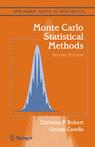yet more questions about Monte Carlo Statistical Methods

Posted in Books, Statistics, University life with tags , , , , , , , , , , on December 8, 2011 by xi'anAs a coincidence, here is the third email I this week about typos in Monte Carlo Statistical Method, from Peng Yu this time. (Which suits me well in terms of posts as  I am currently travelling to Provo, Utah!)

I’m reading the section on importance sampling. But there are a few cases in your book MCSM2 that are not clear to me.

On page 96: “Theorem 3.12 suggests looking for distributions g for which |h|f/g is almost constant with finite variance.”

What is the precise meaning of “almost constant”? If |h|f/g is almost constant, how come its variance is not finite?

“Almost constant” is not a well-defined property, I am afraid. By this sentence on page 96 we meant using densities g that made |h|f/g as little varying as possible while being manageable. Hence the insistence on the finite variance. Of course, the closer |h|f/g is to a constant function the more likely the variance is to be finite.

“It is important to note that although the finite variance constraint is not necessary for the convergence of (3.8) and of (3.11), importance sampling performs quite poorly when (3.12) ….”

It is not obvious to me why when (3.12) importance sampling performs poorly. I might have overlooked some very simple facts. Would you please remind me why it is the case? From the previous discussion in the same section, it seems that h(x) is missing in (3.12). I think that (3.12) should be (please compare with the first equation in section 3.3.2)$\int h^2(x) f^2(x) / g(x) \text{d}x = + \infty$

The preference for a finite variance of f/g and against (3.12) is that we would like the importance function g to work well for most integrable functions h. Hence a requirement that the importance weight f/g itself behaves well. It guarantees some robustness across the h‘s and also avoids checking for the finite variance (as in your displayed equation) for all functions h that are square-integrable against g, by virtue of the Cauchy-Schwarz inequality.